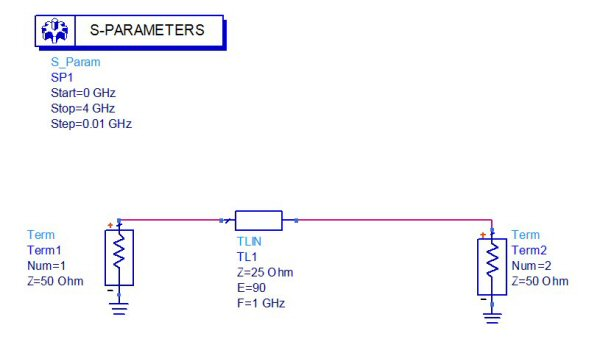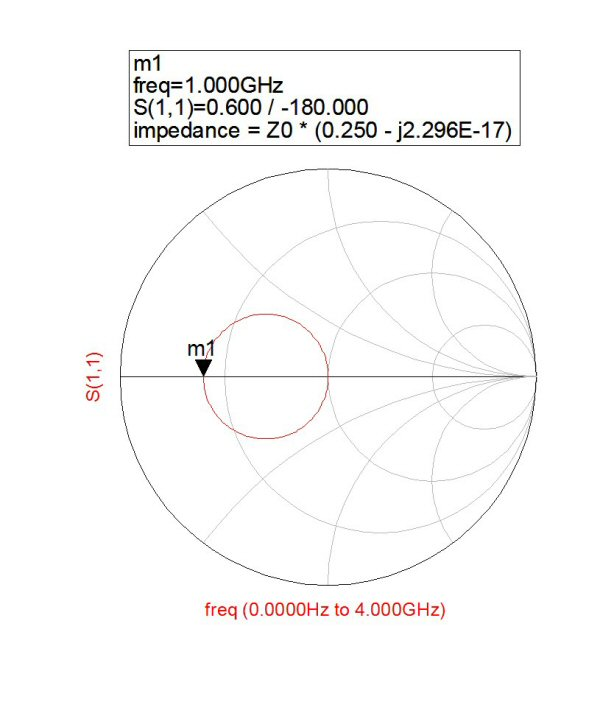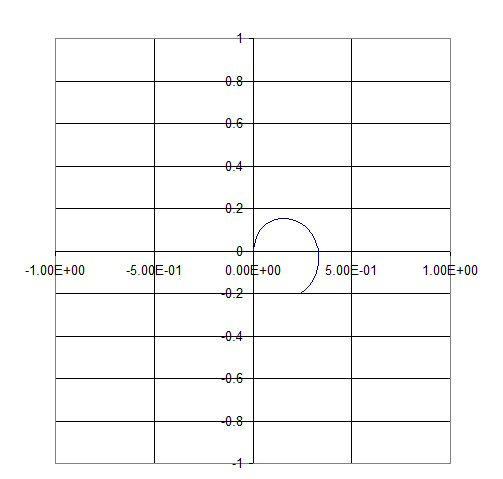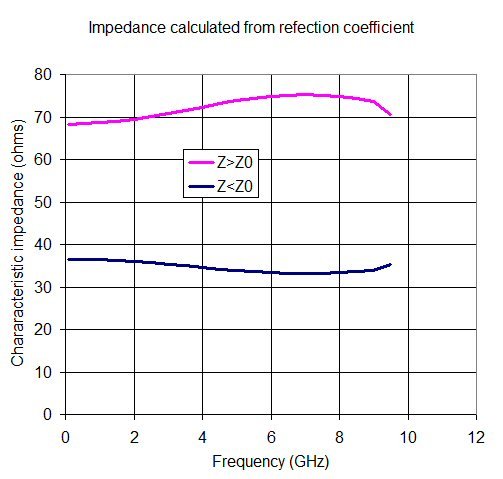# Measuring Characteristic Impedance

This page will tie together some different attempts of measuring characteristic impedance (Z0). About the only thing they have in common is they all use electrical measurements of transmission lines, as opposed to electromagnetic or closed-form simulations. Only one solution (so far) is capable of measuring Z0 over frequency such that dispersion can be observed.

Can you measure Z0 with an ohm-meter?

Measuring Z0 using reflection coefficient

Measuring Z0 on a Smith chart

The Microwaves101 way to measure Z0

Measuring Z0 with time domain reflectometry (separate page)

### Can you measure Z0 with an ohm-meter?

Below is some text we found on the "E-how" web site in 2012, that shows how to test a 50 or 75 ohm cable, the content has since been taken down. There is a lot of misinformation on the world wide web, you have to be careful. They sure have a lot of sponsored links though!

1. Set your multimeter to an ohms scale around 100 ohms. A higher scale works, but avoid moving into a scale close to 1k ohms or higher. Coaxial cable is typically rated to operate at 50 or 75 ohms so the scale should be at or greater than these ratings.

2. Disconnect both ends of the cable from their connections.

3. Test the length of the cable by placing a multimeter probe on each end of the cable, touching the center wire emerging from the coaxial connector.

4. Read the multimeter display. A reading around the 50 ohm or 75 ohm rating of the cable indicates that the cable is working properly across its length. A reading of infinite resistance indicates a break somewhere along the the inside of the cable. A reading of zero indicates a potential short between the inner cable and its shield.

There is no better way to say this, than "what an idiot!" Bad advice is continuous, we won't provide any more of it.

What is this thing called characteristic impedance, also known as "Z-zero"? Let's start with a common misconception. If you have a 50-ohm coax cable (or microstrip line or any other 50 ohm transmission line) that isn't connected to anything on either end, and connect an ohmmeter across it, you might expecting to read 50 ohms if you were new to microwave engineering. But you'll measure an open circuit (unless something is loading the opposite end which means you didn't follow our simple instructions...) nowhere near fifty ohms. What's going on?

But wait, Jens from Denmark wants you to know that the above statement is not 100% technically correct:

On the new page for characteristic impedance, you start out by claiming that you cannot measure anything if you connect an ordinary ohmmeter to a 50 ohm cable or microstrip. This is simply because the line is too short for the meter to get the reading. The meter may take about 1 second to get a stable reading. If you take a loss free transmission of say c x 1.5 (about 4.5 x exp(8) meters!) you will get a reading that lasts about 3 seconds. You may have some practical problems with this experiment though. The line will wind the equator about 11.25 times. And getting it lossless will also be difficult.

Thanks, and let us know when you have that cable in place so we can perform the measurement!

And now an opposing viewpoint from Raphael...

I believe Jens is somehow wrongly deriving is conclusion from the wave propagation phenomena. As I see it, assuming you could make the experiment, you would never see (let's say) 50 Ohm on your meter because traditional multimeters derive resistance from measured voltage and current to display the result of V / I and you would never have any current flowing between the two conductors.

The definition of resistance is "the opposition to the flow of electric current" whereas the definition of impedance is "The opposition to an alternating current". Meaning that a 50 Ohm "resistance" behaves the same (offer the same opposition to current) in both direct or alternating current. A 50 Ohm "impedance" on the other hand does not necessarily behave the same in direct or alternating current.

It's not because an impedance has only a real part that's it's necessarily a "resistance" ... it might just still be an "impedance".
Z = R + jX
Z = R

We're inclined to agree with Raphael on this, but we look forward to further discussion! And here comes some more discussion from Mark, who agrees with Jens...

Although a DC measurement will indeed show no current passing through an unterminated coaxial cable, in a short term view (think in nanoseconds) this is incorrect. When you touch your meter leads to the cable, those leads are at a different electrical potential when compared to the electrical potential between the center conductor and the shield. In order to equalize the potential between the test leads and the cable, current MUST flow. After all, you need charge to have an electrical potential and you need a current to create that charge. During the time where the initial energy applied to the cable is still propagating away from the meter, the meter will indeed read 50 ohms. The problem is, as Jens pointed out, you would either need a very fast multimeter or a very long cable to actually see 50 ohms on a display.

To look at it another way, a lossless cable can be approximated as an infinite series of "L" shaped series inductor, shunt capacitor pairs. When you think in terms of series inductors and shunt capacitors, you can easily imagine getting a different reading until all the L's and C's have stabilized at their steady state values.

To state that the meter would always read an open, requires information to travel faster than the speed of light. After all, how can the meter find out if a cable is terminated until it "queries" it with electrons? For the first 100 us, a 100 us long cable is going to look the same to a meter regardless of any open, short, or loaded conditions on the other end. To do otherwise would require information to travel faster than the wave propagating along the cable.

I have trouble with the message that a meter would take a "look" at the impedance in the first nanosecond and give a meaningful result. The operator would wait a few seconds for the measurement, and he would see an open, then eat another bite of his donut. But you have all given us food for thought! - Unknown Editor

### Measuring Z0 from reflection coefficient

This came from Doug. Thanks! We have also seen it written up in the Microwave Office tutorial. By the way, there is a lot of confusion about the nomenclature Z0, which is the characteristic impedance of a transmission line, and ZT, which is impedance of a termination.  Because Z0 is often matched to ZT, people tend to use the terms interchangably.  Thanks to Parit, we have attempted to clean up teh following text so that it follows convention.

If you measure an unknown cable with unknown Z0, just take the measured S-parameters and renormalize them to whatever value gives you the greatest return loss (i.e., S11< -20 dB or so).  It won't depend on the length (however, it needs to have some measurable length, say greater than lambda/8).   The normalised impedance that gives the lowest value is the Z0 of the unknown transmission line.  For example, ideally, a 75 Ohm transmission line normalised to 75 Ohms will give infinite return loss.

If you display this on a Smith chart, the correct Z0 is the one that gives you the tightest ball in the center. We'll add a picture one of these days. But be aware, it is not possible to see the small effects of dispersion (which can change transmission line impedance slightly over frequency) using this method.

### Measuring Z0 on a Smith chart

If you plot S11 on a Smith chart, for a line length of unknown impedance, it traces a circle, with one point on the circle's circumference at the center of the chart. The diameter of the circle is mathematically related to the line's impedance. If the line were a quarter wave, if you plotted it in frequency up to the quarterwave point it transcribes a half circle. It is easy to figure out the relationship of the line impedance for this case.

Below is a 25 ohm Z0 line "measured" between two fifty ohm ZT terminations:When we plot the reflection coefficient on the Smith chart, you can see the circle that develops to the left of the ZT=50 ohm point. When the line reaches a half wavelength, the circle is complete, then it merely traces over itself as you go further up in frequency. The marker shows an impedance of 0.25 normalized to ZT, or 12.5 ohms (0.25 x 50 ohms).At marker m1 the line forms a classic quarterwave transformer, at this frequency the network appears like a real 12.5 ohm load:

Zin=Z0^2/ZT=25^2/50=12.5

where Z0 is the impedance of the line (acting as a quarterwave transformer), Zin is the impedance looking in to it, and Z0 is the system impedance which terminated both ends of the line.

Solving for Z0 when it is unknown is simply:

Z0=SWRT(Zin*ZT)

The impedance of the line is thus related to the diameter of the circle its reflection coefficient traces over frequency. Just don't make the mistake that the center of the circle represents the transformer's impedance, it does NOT. You have to use the equation shown above to solve for ZT.

By the way, the reflection coefficient magnitude when you are on the real axis to the left of Z0 is:

rho=(Z0-Zin)/(Z0+Zin)

Which in this case is 0.6 (or -4.43 dB if you prefer decibels.

If your reflection coefficient was to the right of Z0 (and on the real axis) the reflection coefficient would be:

rho=(Zin-Z0)/(Zin+Z0).

If you mix these up, the only error will be that the quantity will be a negative number.

### The Microwaves101 way to measure Z0

Below we will discuss an idea we had for measuring characteristic impedance of a transmission line, based on a question that came our way. It is probably not much more than a mathematical exercise, but you never know when it might be useful. If someone gave you a coax cable and didn't know if it was 75 or 50 ohms, this trick might do the job. Its really a calculation of Z0 based on a direct measurement of reflection coefficient. And it is fully capable of measuring dispersion, or that property of microstrip and other not-completely-TEM transmission lines where Z0 changes with frequency (at least in theory!) Here's the premise:

You don't need a full half-wave line to trace out the circle to find its diameter... to determine the diameter of a circle all you need is three points. The circumscribed circle of the triangle they form is easy to calculate, you can look up the formula on Wikipedia. Now you can measure the characteristic impedance of a line from three frequency points of S11 magnitude and angle!

We made an Excel sheet that makes the "diameter" calculation from each set of three successive frequency points. Then it converts to impedance versus frequency. The Excel file is called Impedance Calculator 101.xls, look for it here.

There are possible errors that need to be minimized for this technique to produce an accurate assessment of Z0. If there are parasitics in the measurement (connectors on the ends of a cable of unknown impedance for example) then the calculation could be off. The S-parameters need to be fully deembedded for best results. You will see from the plot that the calculated impedance varies over frequency. Perhaps the lowest error occurs at the lowest frequency, if someone else wants to speculate on this we'd like to hear from you!

There are two solutions to the math. We didn't figure out how to make the spreadsheet smart enough to choose the correct one, but it is easy to pick the right one. If the circle is to the left of center, Z0<ZT. To the right, Z0>ZT.

#### Example 1

We were given reflection coefficient from an electromagnetic simulation of a transmission line. Below it is plotted (by the spreadsheet) from zero to 10 GHz. The circle is to the right side of the origin, so the transmission line must be greater than 50 ohms. Sorry, we were too lazy to plot the data on an actual Smith chart!Now here's what the spreadsheet comes up with for Z0: the characteristic impedance of the line is somewhere between 69 and 75 ohms. A 69 ohm transformer would make the load look like a 95.2 ohm load, or a reflection coefficient of 0.311, which is right about where that circle crosses the X-axis.Why does the impedance vary over frequency? It probably doesn't... this is "measurement error" due to parasitics in the electro-magnetic simulation.

Got some information on measuring characteristic impedance? Send it our way. please!

Author : Unknown Editor